Courses
Courses for Kids
Free study material
Offline Centres
More

# Angle and Tangent Properties of a CircleLast updated date: 27th Nov 2023
Total views: 98.4k
Views today: 0.98k## An Overview of Angle Properties of Circle

The circle is one of the most interesting and important chapters when it comes to geometry. Being an important elementary figure in the field of geometry, circles have many properties associated with them. These properties have great theoretical importance and also have interesting applications.

In this lesson, we will learn about some interesting properties of a circle. We will cover the cyclic and tangent properties of a circle and their theorems along with angle properties of circle questions and answers.

## Cyclic Properties of Circle

Property 1: The angle inscribed in a semicircle is a right angle.

The angle subtended by a semicircle measures 90 degrees. In other words, this property states that if you join the endpoints of a semicircle on a third point somewhere on the circumference of the circle, the angle inscribed would be a right angle.Reference image for property 1

Property 2: Inscribed angles subtended by the same arc are equal.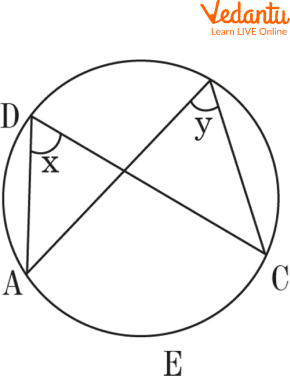Inscribed Angles x and y

In the figure shown above, the arc AEC subtends two inscribed angles $\angle x$ and $\angle y$. Therefore, these two angles will be equal.

Property 3: Central angles subtended by arcs of the same length are equal in measure.

If there are two arcs in a circle that are equal in length, the central angles subtended by them are equal.Reference image for property 3

Property 4: The central angle of a circle is twice any inscribed angle subtended by the same arc.Reference image for property 4

As expressed in the image above, the central angle subtended by the arc is $2 \alpha$, and the inscribed angle subtended by the same arc is $\alpha$.

Property 5: Supplementary opposite angles

The opposite angles of a cyclic quadrilateral are supplementary. In other words, the sum of the measures of the opposite angles of a quadrilateral that is inscribed in a circle is equal to 180 degrees. In the figure below, $\angle \mathrm{A}+\angle \mathrm{C}=180^{\circ}$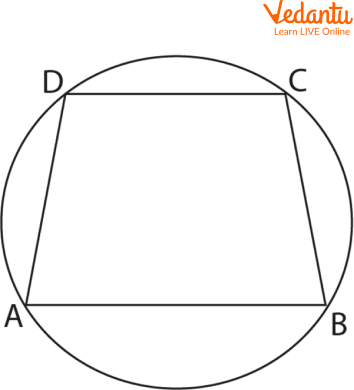Reference image for property 5

## Tangent Properties of Circle

Property 1: A tangent to a circle is perpendicular to the radius drawn to the point of tangency.

If AB is a tangent that has P as a point of contact on the circle with centre O, segment OP will be perpendicular to the tangent AB.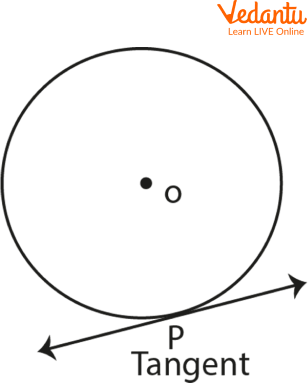Reference image for property 1

Property 2: When two segments are drawn tangent to a circle from the same point outside the circle, the segments are equal in length.Reference image for property 2

## Solved Examples

Below are some angle properties of a circle questions and answers:

Q 1. In the figure given below, if the $\angle A D C=70^{\circ}$, what is the measure of $\angle A B C$?Example 1

Ans: We know that the angle subtended by an arc is twice the angle subtended by it on the circumference.

From the figure, we know that

$\angle \mathrm{ADC}= 70^{\circ}$

So, we get

$\angle \mathrm{ABC}=2 \angle \mathrm{ADC}$

It can be written as

$\angle \mathrm{ABC}=2 \angle 70^{\circ}$

So, we get

$\angle \mathrm{ABC}=140^{\circ}$

## Practice Questions

Q 1. The figure below shows a circle passing through points $A, B, C$, and $D$. The angle $\angle B A C=70^{\circ}$. What is the measure of $\angle DBC+\angle DCB$?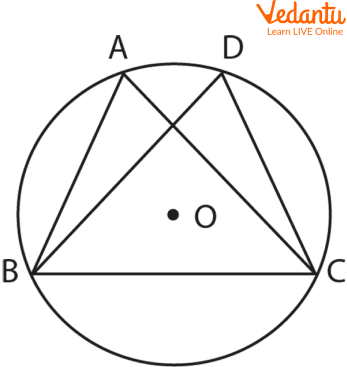Image for Practice Question 1

Ans: $110^{\circ}$

Q 2. In the figure given below, $A C$ is the diameter of the circle. $A B=B C$ and $\angle A E D=118^{\circ}$. Find $\angle D A B$ ?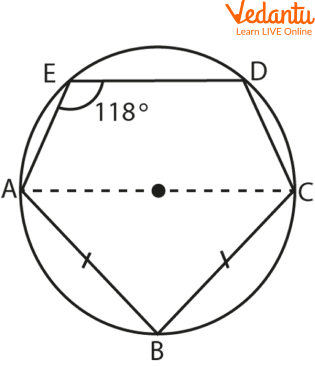Image for Practice Question 2

Ans: $\angle D A B$ = $73^{\circ}$

## Summary

In this article, we focused on one of the most important topics in the chapter on Circles. We learned numerous angle and cyclic properties of circles that helped us in understanding theorems. Not just that but we also covered all the angle and tangent properties of circles. To have better clarity about the application of the properties, we went through the angle properties of a circle worksheet, where we solved some examples using the properties. These properties and theorems are helpful in understanding and solving geometric problems.

## FAQs on Angle and Tangent Properties of a Circle

1.What is the condition for the congruence of two circles?

For two circles to be congruent, both circles need to have radii of equal length.

2.What is the CPCT rule?

CPCT is a short form for Corresponding Parts of Congruent Triangles. The rule means that if two or more triangles are congruent in nature, then all the corresponding angles and sides must be congruent as well.

3. What does SSS mean in geometry?

If all the three sides of one triangle are equivalent to the corresponding three sides of the second triangle, then the two triangles are said to be congruent by SSS rule.